## RootsCamp’s Next Top Model: What is a (statistical) model?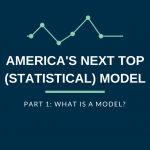# RootsCamp’s Next Top Model: What is a (statistical) model?

Quick Tip

This post is part of a three-part series based on a training given at NOI’s RootsCamp Conference last month. Check out the second part of the series here and part three here.

If you’ve been paying attention to politics in recent years, you’ve probably heard people talk about how a campaign built a model to predict voter turnout.  “Big data” and statistical models have played a growing role in politics and organizing, but how they work remains a mystery to many.  This series will demystify the role that statistical models play in organizing and will introduce some of the concepts involved in building models.

WHAT ARE MODELS FOR?

Statistical models can be used to make inferences and predictions about nearly anything.  They do this by taking the information that we already have, and giving us predictions about the data that we don’t have.Models can predict everything from who will unsubscribe from your organization’s email list, to which movies on Netflix you might like, to who will vote in a given election.  In all of these examples, we feed the model data that we have (data from a political poll for example), and the model gives us predictions about data that we do not have (how voters who did not get surveyed will vote).

There are many different ways to create statistical models — this post introduces some of the concepts that we must think about when creating models.

STARTING SIMPLE: LINEAR REGRESSION

As described above, one easy way of thinking about statistical models is to think of them as tools that take information we have and give us information that we want.  For example, say we know someone’s age — we could build a model that takes this information and gives us an estimate of that person’s height.  This is what’s known as linear regression, and it’s what’s most commonly taught in introductory statistics courses: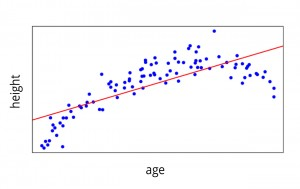Each blue dot in the graph above represents one person.  You can see that the model (the red line) does a reasonably good job of modeling the actual data, but it doesn’t quite pick up the downward trend towards the right side of the graph.  A more complex model might capture the trends in this data even better — we’ll get into that later.

The same general idea can be expanded to include multiple variables.  For example we might know someone’s weight in addition to their age.  This additional information should allow us to build a more accurate model for predicting height.  The 3D chart below demonstrates this relationship.  We can imagine using this same technique with even more dimensions, but it gets a little tricky to draw those charts.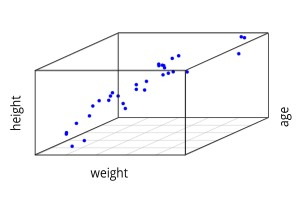THE NEXT STEP: LOGISTIC REGRESSION

Often in politics and advocacy, we are not interested in a continuous variable like height, instead we are frequently interested in binary variables.  Binary variables are measures that fall into one of two outcomes: for example whether or not a particular person votes, whether or not someone supports my candidate, whether or not someone makes a donation, etc.

Linear models don’t work well in these situations.  The chart below shows how age relates to whether or not the individual voted for the Republican or the Democrat.  The line represents the linear regression model that best fits this data.  The line tells us the estimated probability that a randomly chosen person will vote for the Republican candidate if all we know is the person’s age.  You can see that the line goes below zero towards the left side of the chart and goes above one on the right side.  These values don’t make sense and point to one of the problems with using this type of model for a binary outcome.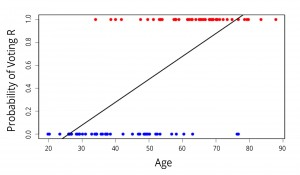Instead of a linear model, one popular option is to use logistic regression to model binary outcomes.  There are more in-depth explanations of the difference between linear and logistic regression HERE and HERE, but the basic idea is that it changes the model from a straight line to an S-shaped line: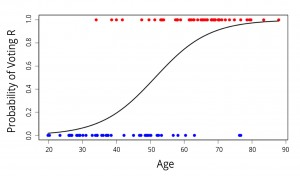As you can see, the logistic model approaches zero and one, but it never goes below zero or above one. This is just one of the reasons that logistic regression works well in predicting binary outcomes.

That’s it for now!  In the next two parts of this series, we will discuss more about how to build models and how to evaluate the quality of a model.

## Written By